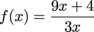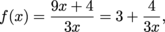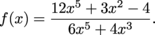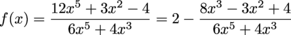Study Guide

# Horizontal, Slant, and Curvilinear Asymptotes

## Horizontal, Slant, and Curvilinear Asymptotes

We've talked about vertical asymptotes where y runs off forever, but whoever said x can't ride off into the sunset (or the negative sunset), too? In this section we'll talk about other types of asymptotes and give tips on how to find their location.

• ### Horizontal Asymptoes

If f(x) is a function and there's some number L withwe draw a dashed horizontal line on the graph at height y = L. This line is called a horizontal asymptote. As x approaches ∞, the function f(x) gets closer to L, in the graph the function gets closer to the dashed horizontal line.We also draw a horizontal asymptote at y = L ifThen as x approaches -∞, or as we move left on the graph, the function f(x) will approach the dashed horizontal line.It's fine for a graph to cross over its horizontal asymptote(s). We can have something like this, for example:The important thing is that as x gets bigger (or more negative), the function is getting closer to the horizontal asymptote.

Finding the horizontal asymptote(s) of a function is the same task as finding the limits of a function f(x) as x approaches ∞ or -∞.

The difference is that horizontal asymptotes are drawn as dashed horizontal lines in a graph, while limits (when they exist) are numbers.

• ### Slant Asymptotes

A slant asymptote, also known as an oblique asymptote, is an asymptote that's a straight (but not horizontal or vertical) line of the usual form y = mx + b (in other words, a degree-1 polynomial). A function with a slant asymptote might look something like this:If a function f(x) has a slant asymptote as x approaches ∞, then the limitdoes not exist, because the function must grow without bound to stay close to the slant asymptote.

• ### Curvilinear Asymptotes

A curvilinear asymptote is an asymptote that's a curve. As x approaches ∞ (or -∞), the function will approach the curve. For example, we could have a function that gets closer to the parabola y = x2 as x approaches ∞ or -∞:• ### Finding Horizontal/ Slant/ Curvilinear Asymptotes

Sometimes when a function has a horizontal asymptote, we can see what it should be.

### Sample Problem

Let f(x) = 4-x. Then as x approaches ∞ the function f approaches 0; there's a horizontal asymptote at y = 0. The function approaches this asymptote as x approaches ∞.

As x approaches -∞ the function f grows without bound, and therefore does not approach the asymptote, y = 0.

Sometimes it's a little challenging to see what the asymptote should be, but we're up for it. These asymptotes often appear when drawing rational functions. First we'll go through the guaranteed method that will tell us what type of asymptote we have and what it is, and then we'll show a shortcut for finding horizontal asymptotes.

### The Polynomial Long Division Method

The guaranteed method is polynomial long division. This method will probably take some time, but it will get the answer.

When finding horizontal / slant / curvilinear asymptotes of a rational function, we do long division to rewrite the function. We throw away the remainder, and what is left is our asymptote. If we're left with a number, that's a horizontal asymptote (and remember, 0 is a perfectly good number). If we're left with a line of the form y = mx + b (in other words, a degree-1 polynomial), that line is our slant asymptote.

If we're left with anything else, it's a curvilinear asymptote.

The reason we throw away the remainder is that it will be a rational function whose numerator has a smaller degree than the denominator, and we know the limit of such a function as x approaches ∞ is 0.

A rational function will approach its horizontal / slant / curvilinear asymptote when x is approaching ∞ and when x is approaching -∞.

### The Shortcut

Congratulations; we've survived the long division. Our reward is a shortcut for finding horizontal asymptotes of rational functions. A horizontal asymptote will occur whenever the numerator and denominator of a rational function have the same degree.

Find the horizontal asymptote of the function.

If we do long division, we findso the horizontal asymptote is y = 3.

### Sample Problem

Find the horizontal asymptote of the functionIf we do long division, we findTherefore, the horizontal asymptote is y = 2.

Notice a pattern? We divide the leading term of the numerator by the leading term of the denominator, and that gives us the horizontal asymptote. That's it.

To summarize:

If a rational function has...

• a smaller degree polynomial in the numerator than in the denominator, that function will have a horizontal asymptote at 0. All done!
• the same degree polynomial in the numerator as in the denominator, that rational function has a horizontal asymptote which we can find by dividing leading terms only.
• a numerator one degree larger than the denominator, that rational function has a slant asymptote, which we can find by long division.
• none of the above, the function has a curvilinear asymptote, which we can find by long division.